# KSEEB Solutions for Class 6 Maths Chapter 5 Understanding Elementary Shapes Ex 5.2

Students can Download Chapter 5 Understanding Elementary Shapes Ex 5.2 Questions and Answers, Notes Pdf, KSEEB Solutions for Class 6 Maths helps you to revise the complete Karnataka State Board Syllabus and score more marks in your examinations.

## Karnataka State Syllabus Class 6 Maths Chapter 5 Understanding Elementary Shapes Ex 5.2

Question 1.
What fraction of a clock wise revolution does the hour hand of a clock turn through, When it goes from
Solution:
We may observe that in 1 complete clock Wise revolution the hour hand will rotate by 360’
a) 3 to 9
When the hour hand goes from 3 to 9 clock wise, it will rotate by 2 right angles or 180°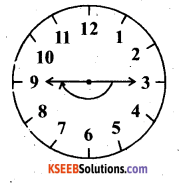b) 4 to 7
When the hour hand goes from 4 to 7 clock wise, it Will rotate by 1 right angle or 90°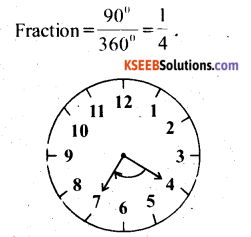c) 7 to 10
When the hour goes from 7 to 10 clock wise it will rotate by 1 right angle or 90°d) 12 to 9
When the hour hand goes from 12 to 9 clock wise it rotate by 3 right angles or 270°e) 1 to 10
When the hour hand goes from 1 to 10 clock wise it rotate by 3 right angles or 170°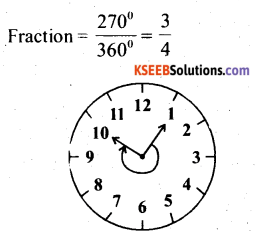f) 6 to 3
When the hour hand goes from 6 to 3 clock wise, it will rotate by 3 right angles or 270°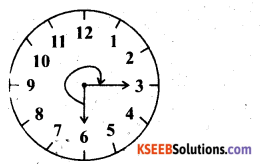Question 2.
Where will the hand of a clock stop if it-
In 1 complete clock wise revolution the hand of a clock will rotate by 360°
Solution:
a) Starts at 12 and makes $$\frac{1}{2}$$ of a revolution, clockwise?
If the hand of the clock start 12 and makes $$\frac{1}{2}$$ of a revolution, clockwise, it will rotate by 180° and hence, it will stop at 6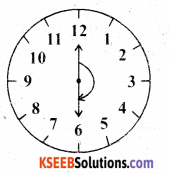b) Starts at 12 and makes $$\frac{1}{2}$$ of a revolution, clockwise?
If the hand of the clock starts at 2 and makes $$\frac{1}{2}$$ of a revolution clock wise, then it will rotate by 180° and hence, it will stop at 8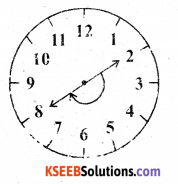c) Starts at 5 and makes $$\frac{1}{4}$$ of a revolution, clockwise?
If the hand of the clock starts at 5 and makes of a revolution clockwise than it will rotate by 90° and hence it will stop at 8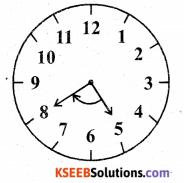d) Starts 5 makes $$\frac{3}{4}$$ of a revolution Clock wise?
Solution:
If the hand of the clock starts at 5 and makes of a revolution clockwise, than it will rotate by 270° and hence. it will stop at 2.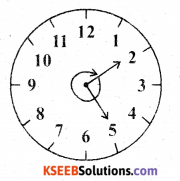Question 3.
Which direction will you face if you start facinga) East and make $$\frac{1}{2}$$ of a revolution clockwise ?
If we start facing east and make $$\frac{1}{2}$$ of a revolution clockwise, than we will face the west direction.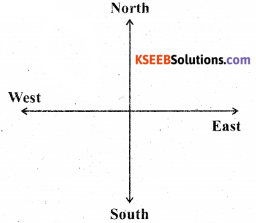b) East and make $$1 \frac{1}{2}$$ of a revolution clockwise?
If we starts facing east and make $$1 \frac{1}{2}$$ of a revolution clock wise, than we will face the West directionc) West and makes at $$\frac{3}{4}$$ of a revolution anti-clock wise ?
If we starts facing west and make of a revolution anti – clockwise, than we face the north direction.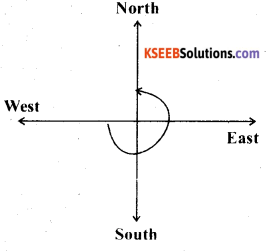d) South and make one full revolution (should we specify clockwise or anti – clockwise for this last question? Why not?
There is no need to specify clockwise or anti-clockwise in the last question as turning by one full revolution (i.e., two straight angles) make us to reach the original position.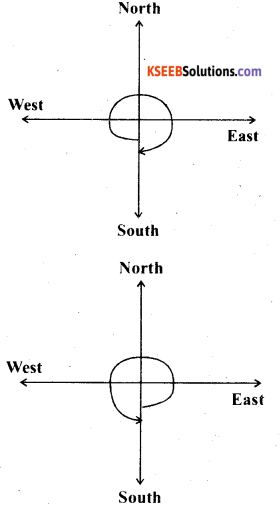In case of revolving by 1 complete round the direction in which we are revolving does not matter in both cases clockwise or anti – clock wise, we will be back at our initial position.

Question 4.
What part of a revolution have you turned through if you stand facing
Solution:
If we revolve one complete round in either clock wise or anti-clockwise direction, then we will revolve by 360° and the two adjacent directions will be at 90° or $$\frac{1}{4}$$ of a complete revolution away from each other

a) east and turn clock wise to face north?
If we start facing east and than turn clockwise to lace north, than we have to make revolutionb) South and turn clockwise to face east?
If we starts facing south and turn clock wise to face east than we have to make $$\frac{3}{4}$$ of a revolution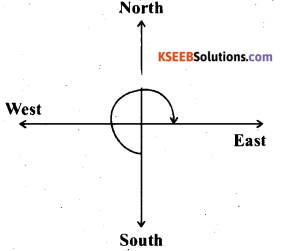c) West and turn clock wise to face east ?
If we starts facing west and turn dock wise to face east, then we have to make $$\frac{1}{2}$$ of a revolution.Question 5.
Find the number of right angles turned through by the hour hand of a clock when it goes from
Solution:
a) 3 to 6
The hour hand of a clock revolves by 360° of 4 right angles in 1 complete round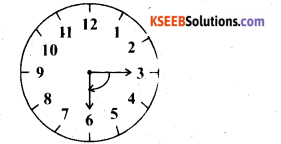b) 2 to 8
The hour hand of a clock revolves by 180° or 2 right angles when it goes from 2 to 8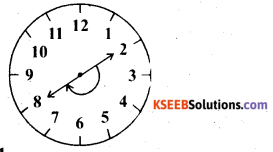c) 5 to 11
The hour hand of a clock revolved by 180° or 2 right angles When it goes from 5 to 11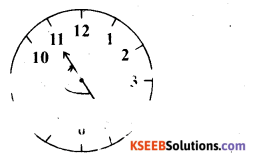d) 10 to 1
The hour hand or a clock revolves by 90° or 1 right angle when it goes from 10 to 1e) 12 to 9
The hour hand of a clock revolves by 2700 or 3 right angles When it goes from 12 to 9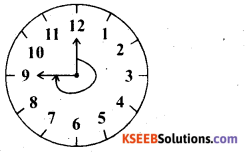f) 12 to 6
The hour hand of a clock revolves by 180° or 2 right angles When it goes from 12 to 6.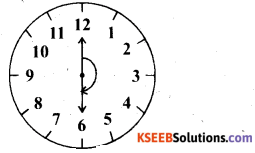Question 6.
How many right angles do you make if you start facing
Solution:
If we revolve one complete round in either clock wise direction, then we will revolve by 360° or 4 right angles and the two adjacent direction will be at 90° or I right angles away from each other.
a) South and turn clock wise to west?
If we start facing south and turn clock wise to west then we make 1 right angle.
clockwise to west then we mu. iv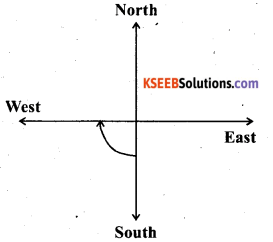b) north and turn anti-clock wise to east ?
If we start facing north and turn anti clockwise to east then we make 3 right angles.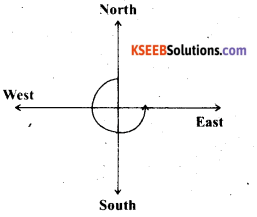c) West and turn to west?
If we starts facing west and turn to west then we make 1 complete round or 4 right.d) South and turn to north?
If we starts facing south and turn to we make right angles.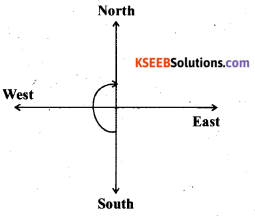Question 7.
Where will the hour hand of a clock stop if it starts
Solution:
In 1 complete revolution ( Clockwise or anti – clock wise) the hour hand of a clock will rotate by 360° or 4 right angles.

a) from 6 and turns through 1 right angle?
If the hour hand of a clock starts from 6 and turns through 1 right angle, then it will stop at 9b) From 8 and turn through 2 right angles?
If the hour hand of a clock starts from 8 and turned through 2 right angles, then it will stop at 2.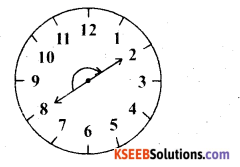c) From 10 and turned through 3 right angles?
If the hour hand of a clock starts from 10 and turns through 3 right angles then it will stop at 7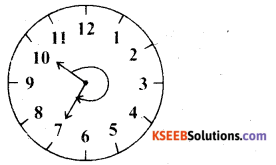d) From 7 and turns through 2 straight angles?
If the hour hand of a clock starts from 7 and turns through 2 straight angles, then it will stop at 7 .error: Content is protected !!